# Restrictions When Calling Functions in Oracle

## Restrictions When Calling Functions in Oracle

In this article, I am going to discuss Restrictions When Calling Functions in Oracle with Examples. Please read our previous article where we discussed the Advantages of User Defined Functions in Oracle.

##### Restrictions When Calling Functions in Oracle

Until now we understood how to use the user-defined functions in select statements in select clauses and in the where conditions. Now, let’s try to understand restrictions when calling functions from SQL expressions.

• The functions must be stored in the database. We can even use the functions without storing in the database. We will learn that when we start learning about packages.
• The user-defined functions accept only IN parameters with valid SQL data types, not PL/SQL-specific types (Record, table, Boolean). So, in order to use the user-defined functions it should only contain only IN parameter.
• Return a valid SQL data type, not the PL/SQL – specific data types.
• When calling functions in SQL statements the parameters must be specified with positional notation but this is before the 11g version of the Oracle database. But now we can call the function with the naming convention.
• You must own the function or have the execute privilege.
• Cannot be used in check constraints. (Create table/ alter table).
• Cannot be used as a default value for a column.

Controlling side effects when calling functions from SQL expressions.

##### Functions called from:
• A SELECT statement cannot contain DML statements. In order to use the function in the select statement the function does not contain the DML Statements.
• An update or delete statement on a table cannot query or contain DML on the same table. This gives us the error called a mutating table.
• SQL statements cannot end transactions that cannot execute COMMIT or ROLLBACK operations.
• Calls to sub-programs that break these restrictions are also not allowed in the functions.

To understand this much better let’s try to do an exercise and see how this restriction works.

```create or replace function get_sal_tax
(p_sal number)
return number
is

begin
commit;

if p_sal<5000 then
return p_sal* (10/100);
else
return p_sal* (15/100);
end if;

end;
```

From the above statement, we can see the function name is get_sal_tax. This is the same as the previous function but we have added only a commit in the function. The code of the function is the same as the previous one. Let’s go ahead and compile this function.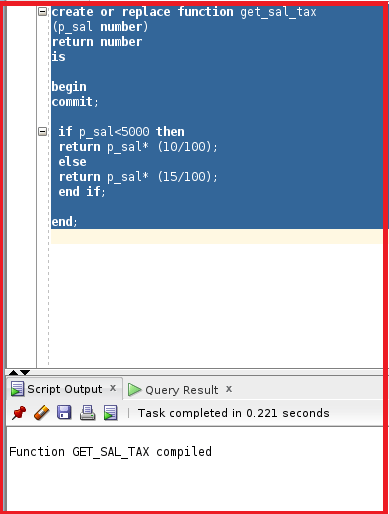So, the function is now compiled. Let’s now try to run the select statement and see how the function works as we have added compile in the function.

Select employee_id, first_name, salary, get_sal_tax(salary) from employees where get_sal_tax(salary)>2000 and order by get_sal_tax(salary);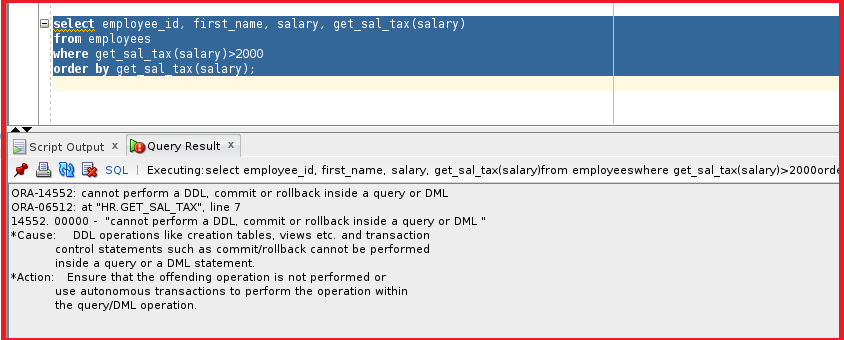We can see the error mentioning” Cannot perform a DDL, commit or rollback inside a query or DML”. So, this means if the select statement is calling any function, then the PL/SQL block contained in the function should not contain commit or rollback. But without the select statement by declaring variable it works. Let’s take the below example.

```declare
v number;
begin
v:=get_sal_tax(5000);
DBMS_OUTPUT.put_line(v);
end;
```

We have declared a variable ‘v’ with a number as a datatype. The return value is now assigned to v. Let’s try to execute this pl.sql block.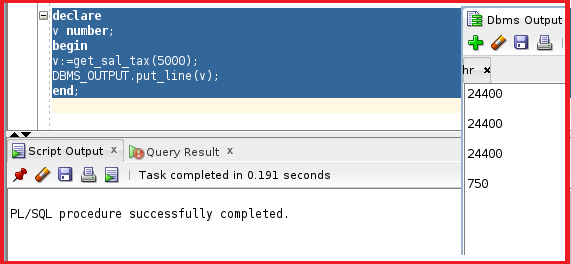The PL/SQL block is executed and we can see the tax is 750 in DBMS OUTPUT. Let’s try another example. Let’s try to use the insert statement in the function and try to execute the function in the select statement or in the separate PL/SQL block.

```create or replace function get_sal_tax
(p_sal number)
return number
is

begin

insert into DEPARTMENTS (DEPARTMENT_ID, DEPARTMENT_NAME)
values (-99,'test');

if p_sal<5000 then
return p_sal* (10/100);
else
return p_sal* (15/100);
end if;

end;
```

In the above function, we have added an insert statement that inserts records into the department table. Let’s try to compile this function.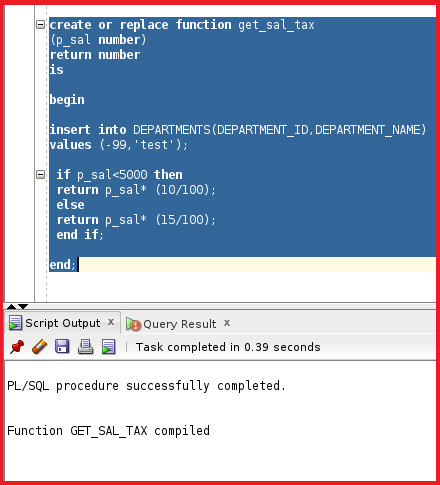So, the function is now compiled. Let’s try to call this function in the select statement and see how this works.

```select employee_id, first_name, salary, get_sal_tax(salary)
from employees
where get_sal_tax(salary)>2000
order by get_sal_tax(salary);
```

Let’s try to execute this select statement.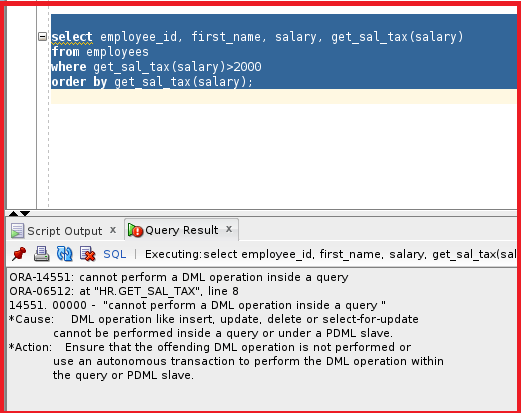So, we are getting the same error which showing cannot perform a DML operation inside a query. But we can execute the function by declaring the variable and calling the function as below.

```declare
v number;
begin
v:=get_sal_tax(5000);
DBMS_OUTPUT.put_line(v);
end;
```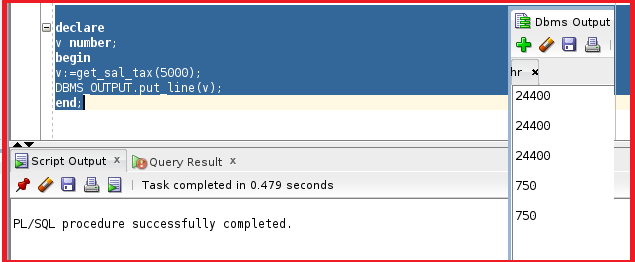Let’s now check if the record is inserted in the departments table or not.

select * from departments where department_id=-99;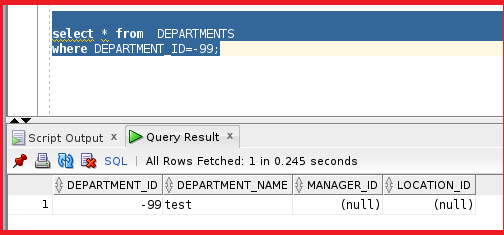So, we can see the record as well in the departments table. We use the function to return a value. We don’t use the function to insert records. We can also drop the function in the below drop command.

drop function get_sal_tax;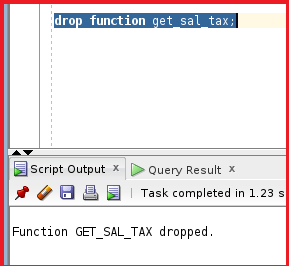So, the function is now dropped. This is how we play with the functions. We have learned about how to create functions and how to use user-defined functions and restrictions with functions.

In the next article, I am going to discuss Packages in Oracle with Examples. Here, in this article, I try to explain Restrictions When Calling Functions in Oracle with Examples. I hope you enjoy this Restrictions When Calling Functions in Oracle article.